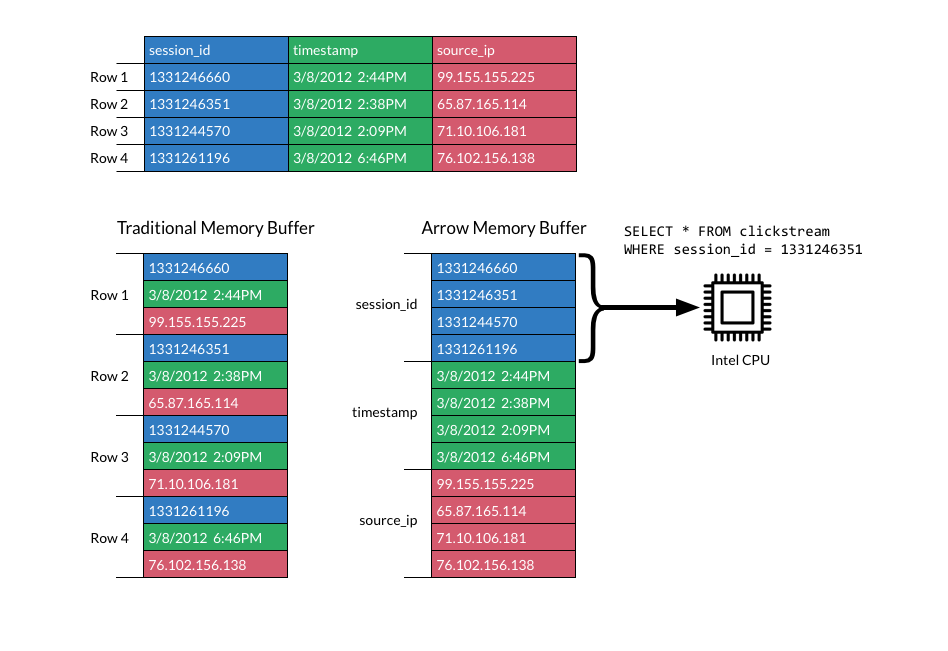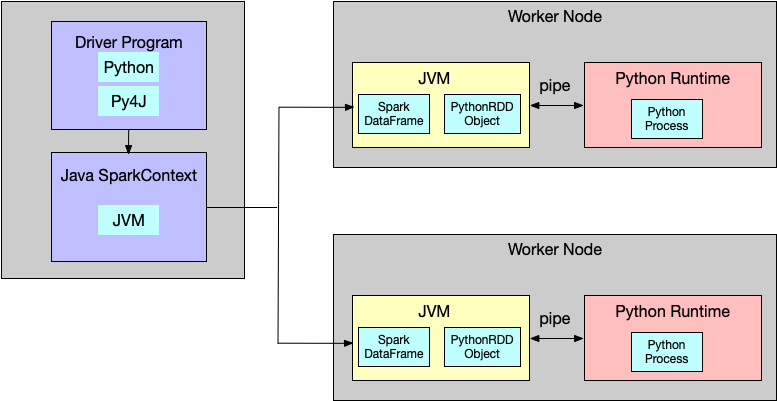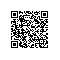# 使用Apache Arrow助力PySpark数据处理

Apache Arrow从Spark 2.3版本开始被引入，通过列式存储，zero copy等技术，JVM 与Python 之间的数据传输效率得到了大量的提升。本文主要介绍一下Apache Arrow以及Spark中的使用方法。

# 列式存储简介# Apache Arrow

Apache Arrow在官网上是这样定义的，Apache Arrow是一个跨语言、跨平台的内存数据结构。从这个定义中我们可以看到Apache Arrow与Apache Parquet以及Apache ORC的区别。Parquet与ORC设计的目的针对磁盘数据，在列存储的基础上使用了高效率的压缩算法进行压缩，比如使用snappy、gzip和zlib等算法对列数据进行压缩。所以大部分情况下在数据读取的时候需要首先对数据进行反压缩，并有一定的cpu使用损耗。而Arrow，作为在内存中的数据，并不支持压缩(当然写入磁盘是支持压缩的)，Arrow使用dictionary-encoded来进行类似索引的操作。

# Spark与Apache Arrowif use_arrow:
try:
from pyspark.sql.types import _check_dataframe_convert_date, \
_check_dataframe_localize_timestamps
import pyarrow
batches = self._collectAsArrow()
if len(batches) > 0:
table = pyarrow.Table.from_batches(batches)
pdf = table.to_pandas()
pdf = _check_dataframe_convert_date(pdf, self.schema)
return _check_dataframe_localize_timestamps(pdf, timezone)
else:
return pd.DataFrame.from_records([], columns=self.columns)
except Exception as e:
# We might have to allow fallback here as well but multiple Spark jobs can
# be executed. So, simply fail in this case for now.
msg = (
"toPandas attempted Arrow optimization because "
"'spark.sql.execution.arrow.enabled' is set to true, but has reached "
"the error below and can not continue. Note that "
"'spark.sql.execution.arrow.fallback.enabled' does not have an effect "
"on failures in the middle of computation.\n  %s" % _exception_message(e))
warnings.warn(msg)
raise

    def _collectAsArrow(self):
"""
Returns all records as a list of ArrowRecordBatches, pyarrow must be installed
and available on driver and worker Python environments.

.. note:: Experimental.
"""
with SCCallSiteSync(self._sc) as css:
sock_info = self._jdf.collectAsArrowToPython()
return list(_load_from_socket(sock_info, ArrowStreamSerializer()))

class ArrowStreamSerializer(Serializer):
"""
Serializes Arrow record batches as a stream.
"""

def dump_stream(self, iterator, stream):
import pyarrow as pa
writer = None
try:
for batch in iterator:
if writer is None:
writer = pa.RecordBatchStreamWriter(stream, batch.schema)
writer.write_batch(batch)
finally:
if writer is not None:
writer.close()

import pyarrow as pa
yield batch

def __repr__(self):
return "ArrowStreamSerializer"

 def collect(self):
"""Returns all the records as a list of :class:Row.

>>> df.collect()
[Row(age=2, name=u'Alice'), Row(age=5, name=u'Bob')]
"""
with SCCallSiteSync(self._sc) as css:
sock_info = self._jdf.collectToPython()
return list(_load_from_socket(sock_info, BatchedSerializer(PickleSerializer())))

import pandas as pd

from pyspark.sql.functions import col, pandas_udf
from pyspark.sql.types import LongType

# Declare the function and create the UDF
def multiply_func(a, b):
return a * b

multiply = pandas_udf(multiply_func, returnType=LongType())

# The function for a pandas_udf should be able to execute with local Pandas data
x = pd.Series([1, 2, 3])
print(multiply_func(x, x))
# 0    1
# 1    4
# 2    9
# dtype: int64

# Create a Spark DataFrame, 'spark' is an existing SparkSession
df = spark.createDataFrame(pd.DataFrame(x, columns=["x"]))

# Execute function as a Spark vectorized UDF
df.select(multiply(col("x"), col("x"))).show()
# +-------------------+
# |multiply_func(x, x)|
# +-------------------+
# |                  1|
# |                  4|
# |                  9|
# +-------------------+

# Load necessary libraries
from pyspark.sql.functions import pandas_udf, PandasUDFType
from pyspark.sql.types import *
import pandas as pd
from scipy.optimize import leastsq
import numpy as np

# Create the schema for the resulting data frame
schema = StructType([StructField('ID', LongType(), True),
StructField('p0', DoubleType(), True),
StructField('p1', DoubleType(), True)])
# Define the UDF, input and outputs are Pandas DFs
@pandas_udf(schema, PandasUDFType.GROUPED_MAP)
def analyze_player(sample_pd):
# return empty params in not enough data
if (len(sample_pd.shots) <= 1):
return pd.DataFrame({'ID': [sample_pd.player_id],
'p0': [ 0 ], 'p1': [ 0 ]})

# Perform curve fitting
result = leastsq(fit, [1, 0], args=(sample_pd.shots,
sample_pd.hits))
# Return the parameters as a Pandas DF
return pd.DataFrame({'ID': [sample_pd.player_id],
'p0': [result], 'p1': [result]})
# perform the UDF and show the results
player_df = df.groupby('player_id').apply(analyze_player)
display(player_df)